# What is Amperage?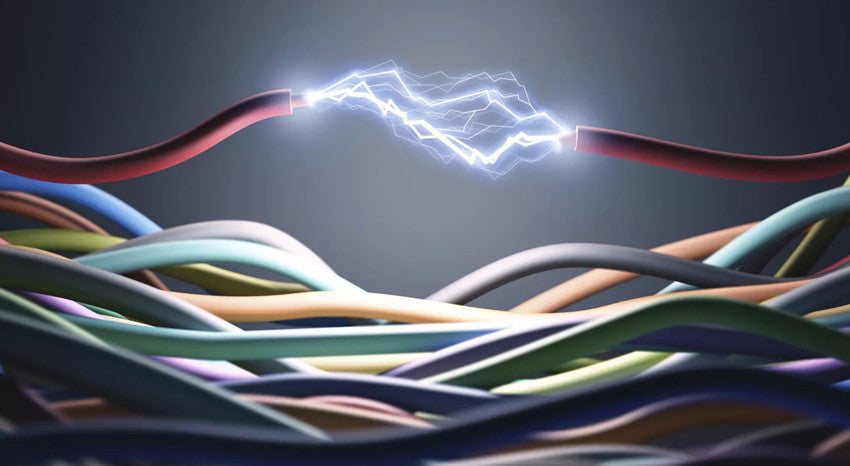Amperage is the current flow of electrons from the negative electrode to the positive electrode in the battery. The current path that Electrons take from Low pressure (ground/Earth) to High Pressure (Live/Hot) in a standard circuit. While this explanation is more scientifically accurate, in Automotive we relate to and more often use the conventional current design in terms of circuits and distinctions.

Let's assume the conventional current flows out of the positive terminal (High Pressure), through the circuit, and into the negative terminal (Low Pressure) of the battery. Because this is commonly used in automotive, we will continue to use these symbols such as diodes and transistors as well as rules such as Right-hand Rules were created using conventional currents. (The right-hand rule is a rule used in mathematics and physics. It is a rule invented by British physicist John Fleming in the late nineteenth century to help his students easily find the direction of the electromotive force generated by a conductor moving in a magnetic field.)## Simple DC Amperage

Today, we want to discuss the Amperage of a DC circuit (Current only flows in one direction). Whether it is Amperage Flow or Current Flow, it is the amount of electric power flowing through the circuit (system) and it creates an electromagnetic force (emf) but we can understand it as the workforce of electricity.  The Amperage typically flows through the motor and pushes the fan on its way from the positive side to the negative side of the circuit. Although the Ampere (Amp) is measured as the flow of electrons over a point over a specific set of time, we will be focusing more on its practical application to test.

Let's take an example, the ampere is the workforce of the battery flowing through the vehicle's circuits and thusly activating what needs to be working, (electrically) The most significant thing is always having the correct electron flow to components. Whether starting a motor, fan, relay, or light-bulb, everything on a vehicle has a specific amperage draw. For example, let's supposed that a fan is designed to operating at 12 volts, 5A, which means the fan is designed to run on a 12-volt circuit with 5 amps.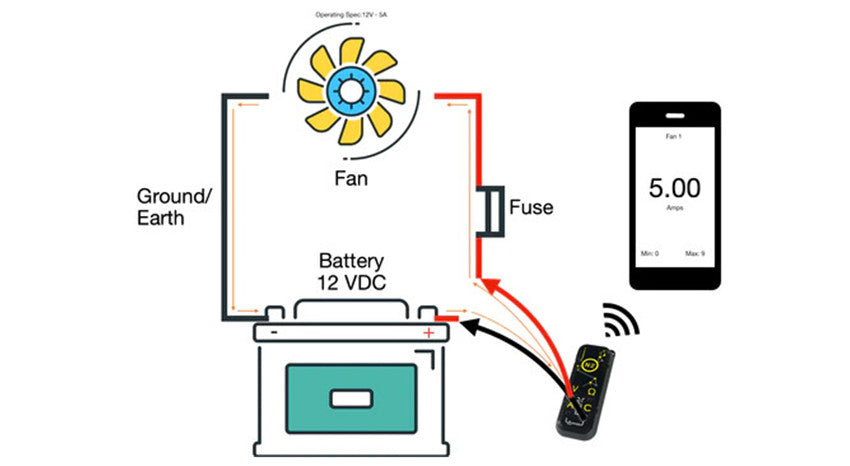## Inrush Current

Most components will only draw as much current as they need to operate at the level they are rated for, however, this does not include a brief moment for something called inrush current. The inrush current is the input surge of current, also known as the Switch-on Surge of current which is the maximum instantaneous amperage drawn by a component when first turned on. In the above, we can see that although the fan is rated for a steady state current draw of 5A (5 amps), it has an inrush of 9 Amps.

This inrush current is the extra workforce that was required to get the motor moving from a complete stop to its standard operating amperage. You can think of this as if you are trying to push a large cart across the workshop, initially you have to give it just a little extra help to get it moving, but once it is moving, momentum and the wheels help to keep it going.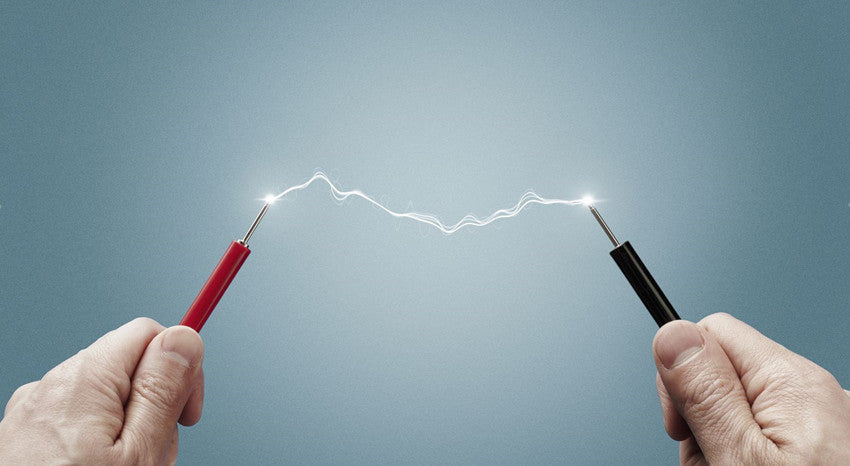## Why do we need to measure Amperage?

Understanding the current consumption of a circuit or component is crucial in automotive because it can help you identify if the current consumption of the component or circuit falls below, at, or higher than the parameters set by the manufacturers. Essentially we can determine the health of a circuit or component based on its amperage draw. If the amperage draw is too low, it may indicate that there is a problem with the power source and that the component is not at full voltage, or current carrying capacity, or the power supply connected to the component is not in specification, or there is resistance on the circuit or a loose connection somewhere. A high amperage draw may indicate that the component is starting to fail, something is preventing the component from working correctly (such as debris in a fan or motor), or there is resistance somewhere in the power feeder. Also if there is amperage on a circuit when there should be none, it can indicate a number of other electrical faults in the system, including a parasitic draw or short circuits.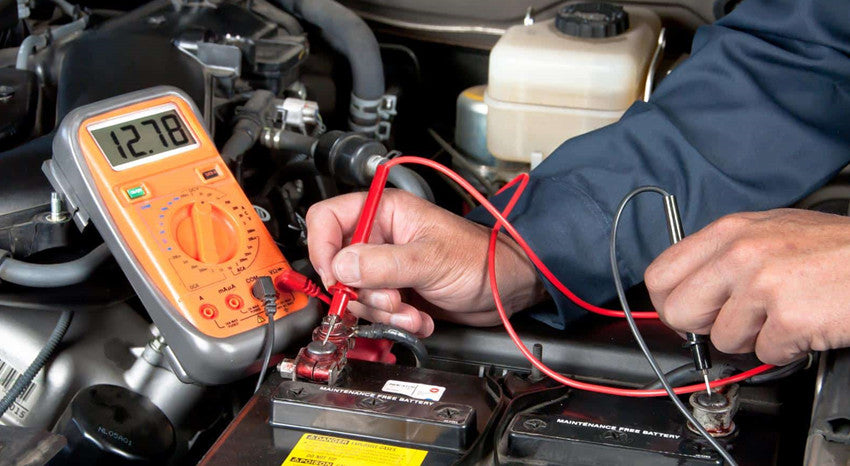## How to measure amperage

There are several ways to measure amperage in a circuit. Firstly, a measurement device like the N2 Neuron is needed because we are measuring the amount of electrons flowing through one point in one second. 6.24 billion (6.24 x 1018) electrons in 1 second = 1 Amp. Once we can measure amperage, there are three methods to find the value of amperage in a circuit or component.

The amperage inline or series test is completed by connecting the measuring device in-line or in series with the circuit (making it part of the circuit) and measuring the amps as the current flows through the device and circuit.  Inline testing although accurate is the most limited option, because in the automotive we typically want to measure the circuit without changing its original operating environment, disturbing any communication or connections to PCM/ECMS in the process of testing and we cannot test (typically) more than 10 amperes at once. Most meters and testing equipment are rated for no more than 10 amps.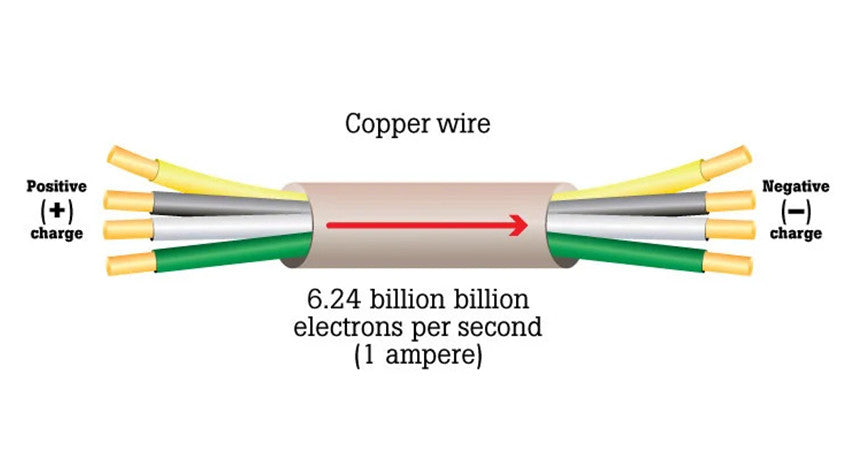Inline amperage testing with a meter on a motor fan circuit conventional flow Inductive (Hall effect) - inductive testing or testing using the specified amperage clamp for the DC circuit (Hall effect is typically used) is a more common method of testing amperage, which allows the circuit to stay intact while providing feedback on the movement of electrons in the circuit. The working mode of the inductive Hall effect clamp is to measure the magnetic field change of the iron core of the clamp. In other words, when electrons in a wire flow, they create a magnetic field. Because we know that the clamp has a known magnetic field and can measure it, when the clamp is placed around the wire and the electrons flowing through it change the magnetic field of the clamp, we can measure the difference and obtain the ampere reading. Although this is a simplified explanation, most clamps will output a millivolt reading and correlate it with an ampere reading, such as 1mv = 1 amp. Also, Also amperage clamps typically come in multiple ranges (low and high amp clamps), because when measuring small amperage, a low-range sensor that can handle magnetic field changes is required, and when reading a high amperage, a sensor that can handle the higher ranges in a change in magnetic fields is needed.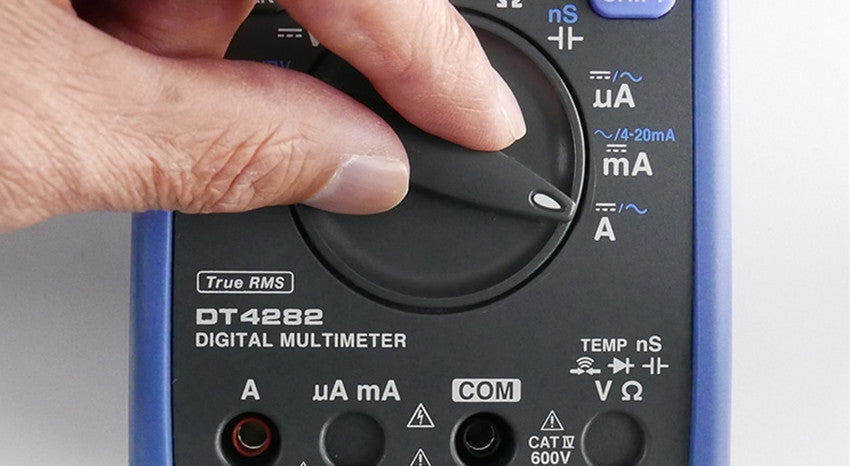## Inductive Ampere Clamp DC Circuit Fan Motor Measurements

Calculation (V=IR) - Another way to measure amperage is by calculation. When using Ohm’s law, we find that voltage equals current multiplied by resistance. In the simplest terms, Ohm's Law tells us that voltage, amperage, and resistance are all related to each other and that a change in one will affect the other two. In the equation V = IR (Volts = Current X Resistance or Ohm’s Law), if we have the other two, we can find any value we want V (Volt), I (Current), or R (Resistance). This brings us back to high school math. Because we know Ohm’s law, if we know the voltage and resistance of the circuit through the voltage drop, we can calculate the amperage value of a circuit.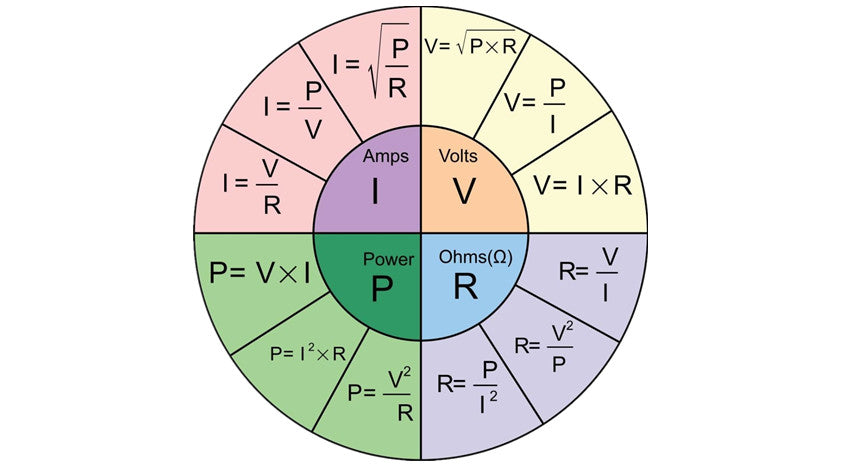This allows us to use a shunt of a known resistance value in series with a circuit, measure the voltage drop across the shunt, and then calculate the amperage. In automotive we can use the fuses in the vehicle to determine the amperage of the circuit. This is extremely helpful for finding parasitic draw without breaking the fuse or disrupting the circuit's natural state. You may ask  how to calculate the amperage draw of a circuit by measuring the voltage drop across a fuse. In this case, we can imagine that some component is drawing the amperage that shouldn’t be and the vehicle is in a key-off position. With that assumption in mind, we can measure the voltage drop at 0.01 volts or 10 MilliVolts, knowing that the resistance rating of a JCase 20 Amp Blue Fuse is approximately 0.006 Ω (Ohm’s) we can draw our calculation as .01 = I X 0 .006 To find I (or Amps) we need to Even Out The Equation by dividing the left side by the number we know on the right (0.01/0.006 = I). When we calculate 0.01/0.006, we find I=1.67, so by simple calculation using Ohm's law, and calculating I or current, we find that this circuit which is supposed to have no draw (or virtually no draw) has a 1.67 A draw which will more than likely drain a battery and is considered a parasitic draw (More on that in future articles). the voltage drop of the fan motor circuit using parasitic draw testing via fuse.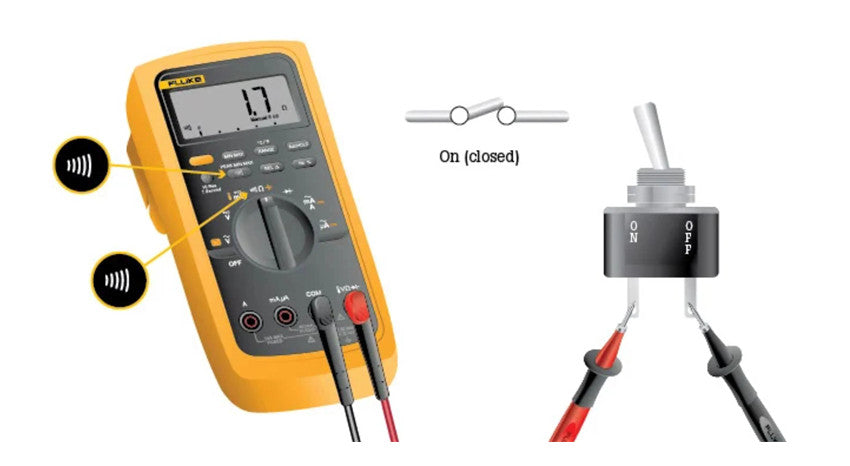## Do I need to know more than amperage?

When it comes to testing the electrical issues on a vehicle it is important to learn as much as possible about a circuit and its operating health or state, so that you can make the best determination on what happens. As mentioned in the previous paragraph regarding Ohm's law calculations, I list a little bit of information and some basic math we can find out more about what is going on in a circuit that our tools (today) can tell us. Knowing amperage in conjunction with Voltage allows us to calculate the resistance, and understanding voltage and resistance allow us to calculate the amperage. It can help us manipulate the data in our hands and lead us toward data intelligence. As we delve deeper into the field of automotive application testing, it will become more apparent that we are living in an information world where we only need a little help to achieve what we want.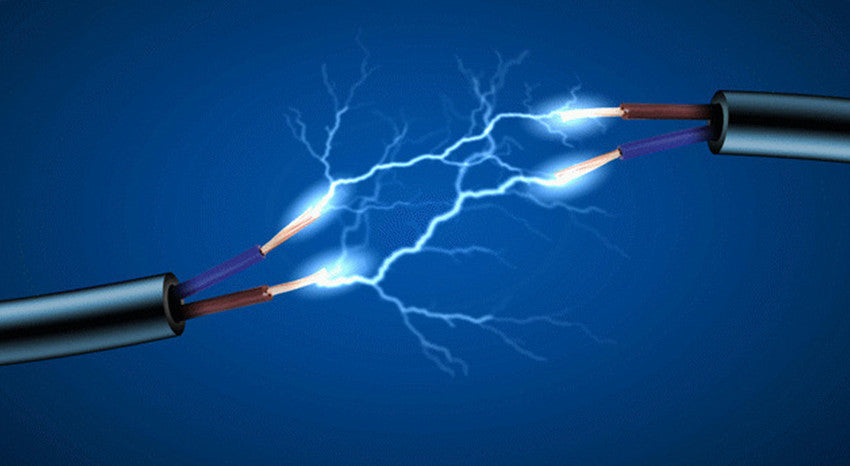## Conclusion

Simplify, amperage is the one-word term for Electrical Current, which is measured in amperes (amps). It describes the amount of electrical charge that is flowing through the system or alternately the maximum amount of electrical current that the system is capable of handling safely. This maximum value can also be referred to as Ampacity, which is a portmanteau of Amperage Capacity. One ampere is the equivalent of a charge of one coulomb (6.241 x 1018 electrons) per second. Thus, if two amperes pass through a given point in an electrical system every second, the amperage of that system is two.

Knowing more about amperage and formulas will help you find problems with electricity in your daily life more easily, and deal with them better.

You have successfully subscribed!
This email has been registered